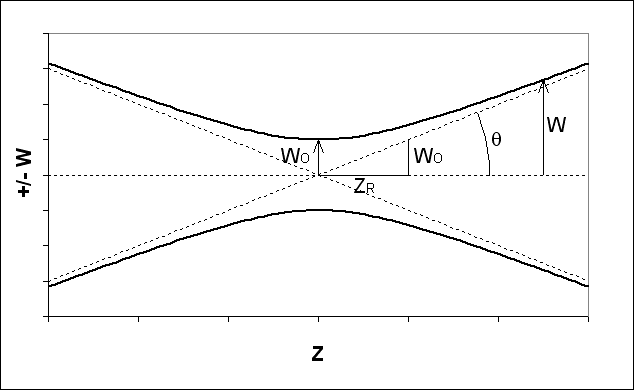# What is a Gaussian beam

Admin
Definition/Summary

A Gaussian beam is an electromagnetic wave, usually a laser beam, with a Gaussian cross-sectional irradiance pattern. The Gaussian irradiance profile results in minimal spreading due to diffraction effects.

The spot size $w$ represents the radius or half-width at which the irradiance is a factor of $1/e^2$ less than the central-axis irradiance.

Equations

For a Gaussian laser beam propagating along the z-axis, the electric field strength is a Gaussian function of the transverse (or radial) coordinate r:

$$E = E_0 \cdot e^{-r^2/w^2}$$

where Eo and w are both functions of z.

It is common practice to work in terms of the irradiance, which is proportional to the square of the electric field, so that

$$I = I_0(z) \cdot e^{- 2 r^2 / w(z)^2}$$

The various parameters of a Gaussian beam are related as follows:

\begin{align*} \theta & = & & \frac{\lambda}{\pi \ w_o} & = & & \sqrt{\frac{\lambda}{\pi \ z_R}} \ & = & & \ \frac{w_o}{z_R} \\ \\ w_o & = & & \frac{\lambda}{\pi \ \theta} & = & & \sqrt{\frac{\lambda \ z_R}{\pi}} \\ \\ z_R & = & & \frac{\pi \ w_o^2}{\lambda} & = & & \frac{\lambda}{\pi \ \theta^2} \\ \\ b & = & & 2 \ z_R \\ \end{align*}

Moreover,

\begin{align*} w(z) & = & & w_o \sqrt{1 + \left(\frac{z}{z_R}\right)^2} \\ \\ R(z) & = & & z + z_R^2/z \ = \ z \left[ 1 + \left( \frac{z_R}{z} \right) ^2 \right] \end{align*}

Extended explanation

Definitions of terms

(SI units for quantities are shown in parantheses)
b = confocal parameter (m)
E = electric field (V/m)
Eo = E at r=0
I, Io = irradiance (W/m2)
r = transverse or radial coordinate (m)
R(z) = radius of curvature of wavefronts (m)
w(z) = spot size (m)
wo = beam waist (m), or spot size at z=0
z = longitudinal coordinate (m)
zR = Rayleigh range
λ = wavelength (m)
θ = divergence half-angle​

Descriptive figure* This entry is from our old Library feature, and was originally created by Redbelly98.

Last edited by a moderator: